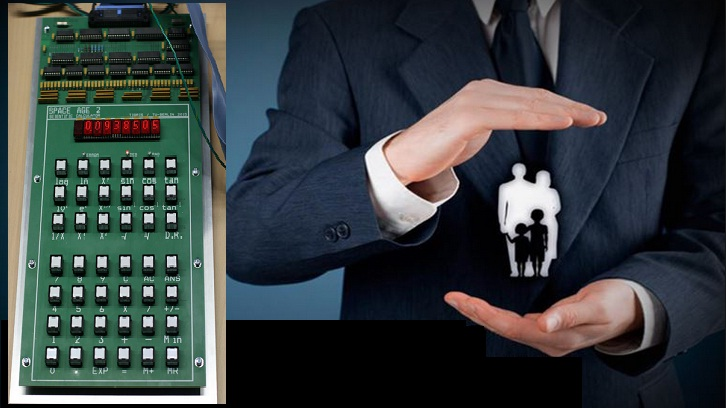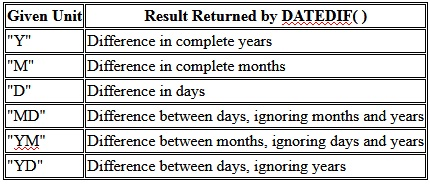# Calculate Your Age and Day of Birth in Excel

•
•
•
•
•
•
•
•
•
•
Sometimes, a person needs the exact age calculation from his / her date of birth to a specific date, for example when he / she applies for a job. The employer demands the required age must not be more that 21 years on the given last date for submitting job application.Calculate your age from your date of birth in MS Excel with Datedif() function

In such situation,  we need to calculate exact date in years, months and days. Because the difference of  a single day will also be considered.
Today, we will learn, how to prepare Microsoft Excel Worksheet for calculating Age in Years, Months and Days with the help of formulas and functions provided in MS Excel. We will also find and display the actual day of birth from the given date of birth for a person.Age Calculator Designed in Microsoft Excel Using DATEDIF Function

### Preparation Of MS Excel Worksheet To Tell your Age from Date of Birth

1. First of all, start Microsoft Excel. Open a new blank workbook in MS Excel.
2. Type the data in MS EXCEL worksheet as shown in above figure.
3. You can perform any formatting on this worksheet with the help of different tools provided by Microsoft Excel like Font, Font Size, Font Color and Borders options.
4. Consider the following worksheet prepared to calculate Age from Date of Birth up to a given date.

### Inserting Formula Using DateDif( ) Built-in Excel Function for Age Calculation from Date of Birth

After entering the data in Microsoft Excel worksheet, it is a time to insert an Excel formula that will use mainly the DateDif( ) function for age calculation from date of birth.
For this purpose, we apply the required age calculation formula in the cell with cell reference D5.
First of all type = then type name of the function DateDif() and type the following formula in its parentheses.

## Formula of Age Calculation From Date of Birth in Excel

Type the following formula without any formatting and without line break in cell with cell reference D5.
=DATEDIF(B5,C5,”Y”)&” Years, ” & DATEDIF(B5,C5,”YM”)&” Months, “ & DATEDIF(B5,C5,”MD”)&” Days”
1. Consider that,  line number 1 in blue color will produce the text 46 Years,
2. Second line with red maroon color will produce the text output  2 Months,
3. Ant the third line will produce text output 12 Days.

### Text Function Used to Display the Day of Birth as shown below in cell E5

=Text(B5,”dddd”)
This function will return the day name on the given date in cell B5.

### DATEDIF( ) Function in MS EXCEL

Microsoft Excel provides a predefined function called DATEDIF(stands for date Difference) which will return the difference between two given dates in the form of years, months or days as required by the user.

### Syntax of DATEDIF( ) Function is as follows:

=DATEDIF(starting_date, ending_date, time_unit)

### The user will provide three arguments to DATEDIF function as follows:

•    starting_date – Starting date in Excel date serial number format.
•    ending_date – Ending date in Excel date serial number format.
•    unit – The time unit to use (years, months, or days). Actually, Y for years, M for months and D for days.

### Example of using DATEDIF( ) Function in Microsoft Excel

=DATEDIF (A1,B1,”Y”)
Let the cell A1 contains starting date 1/3/2016 (3-Jan-2016)
Let the cell B1 contains the ending date  2/4/2017 (4-Feb-2017)
The result of DATEDIFF( ) will be 1. Because there is one complete year btween two given dates.

Therefore,  DATEDIF function will calculate the time interval between a starting_date and an ending_date in years, months, or days. The time unit to return is specified using the unit argument. Unit argument can take the following 6 values in uppercase or lower case, as shown in the following table. Unit argument is provided in the following way:Age Calculation from Date of Birth in Excel Datedif()  function